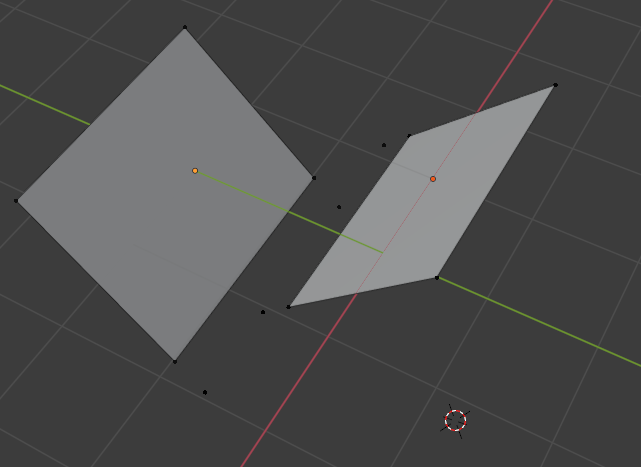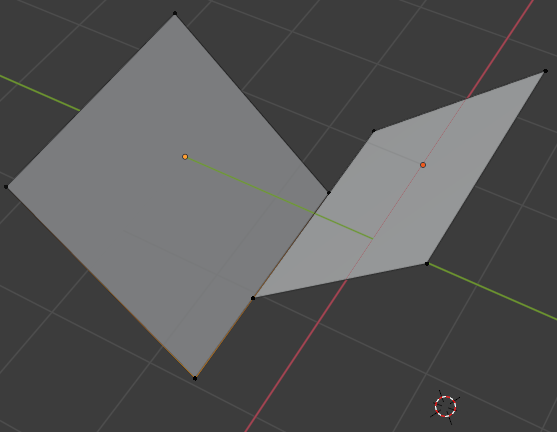# Moving vertex from plane

Hey guys,

I want to move a vertex of a plane, to a specific position (xyz)

how is this done?

i tried to do it with bmesh or the translate method which is only possible in 3d view but this simple task makes me fail all the timeFind the object, find the mesh associated (data), find the vertex you need to change, than just set their new coordinate. You may need to be in edit mode.

``````# List all selected objects with meshes
objs = [o for o in bpy.context.selected_objects if o.type == 'MESH']
for obj in objs:
for meshVertex in obj.data.vertices:
meshVertex.co = [0,0,0] # x, y, z
``````

I use the word “find” because it mostly depends on how you identify this object/vertices to change them.

1 Like

i tried that already but nothing happens:

``````    p1v1 = edge_obj_1.matrix_world @ edge_obj_1.data.vertices.co
p1v2 = edge_obj_1.matrix_world @ edge_obj_1.data.vertices.co
p1v3 = edge_obj_1.matrix_world @ edge_obj_1.data.vertices.co
p1v4 = edge_obj_1.matrix_world @ edge_obj_1.data.vertices.co

p2v1 = edge_obj_2.matrix_world @ edge_obj_2.data.vertices.co
p2v2 = edge_obj_2.matrix_world @ edge_obj_2.data.vertices.co
p2v3 = edge_obj_2.matrix_world @ edge_obj_2.data.vertices.co
p2v4 = edge_obj_2.matrix_world @ edge_obj_2.data.vertices.co

intersect_p1s1 = mathutils.geometry.intersect_line_line(p1v2,p1v4,v1,v2)
intersect_p1s2 = mathutils.geometry.intersect_line_line(p1v1,p1v3,v1,v2)

intersect_p2s1 = mathutils.geometry.intersect_line_line(p2v2,p2v4,v1,v2)
intersect_p2s2 = mathutils.geometry.intersect_line_line(p2v1,p2v3,v1,v2)

print(intersect_p1s1)
print(intersect_p1s2)

print(intersect_p2s1)
print(intersect_p2s2)

p1v1 = intersect_p1s1

edge_obj_1.data.update()
``````

data.update() doesnt help :/. @Debuk what do you think?

You dont write it back to the vertex you just modify eg p1v1.

``````    p1v1 = edge_obj_1.matrix_world @ edge_obj_1.data.vertices.co
p1v2 = edge_obj_1.matrix_world @ edge_obj_1.data.vertices.co
p1v3 = edge_obj_1.matrix_world @ edge_obj_1.data.vertices.co
p1v4 = edge_obj_1.matrix_world @ edge_obj_1.data.vertices.co

p2v1 = edge_obj_2.matrix_world @ edge_obj_2.data.vertices.co
p2v2 = edge_obj_2.matrix_world @ edge_obj_2.data.vertices.co
p2v3 = edge_obj_2.matrix_world @ edge_obj_2.data.vertices.co
p2v4 = edge_obj_2.matrix_world @ edge_obj_2.data.vertices.co

intersect_p1s1 = mathutils.geometry.intersect_line_line(p1v2,p1v4,v1,v2)
intersect_p1s2 = mathutils.geometry.intersect_line_line(p1v1,p1v3,v1,v2)

intersect_p2s1 = mathutils.geometry.intersect_line_line(p2v2,p2v4,v1,v2)
intersect_p2s2 = mathutils.geometry.intersect_line_line(p2v1,p2v3,v1,v2)

print(intersect_p1s1)
print(intersect_p1s2)

print(intersect_p2s1)
print(intersect_p2s2)

edge_obj_1.data.vertices.co = edge_obj_1.matrix_world.inverted() @ intersect_p1s1
edge_obj_1.data.vertices.co = edge_obj_1.matrix_world.inverted() @ intersect_p1s2

edge_obj_2.data.vertices.co = edge_obj_2.matrix_world.inverted() @ intersect_p2s1
edge_obj_2.data.vertices.co = edge_obj_2.matrix_world.inverted() @ intersect_p2s2
``````

thanks Its working, just inverted the matrix because i cannot do it with global coordinates i guess. or is there a way on doing this?No, vertices are stored in modelspace.

1 Like

okay good to know!

it would be great if I could select only the edges instead of switching between object and edit mode :s…

Yeah I see, I just dont know what you want to achieve here, so its not easy to give a good tip on this. In principle this is how blenders works, you select multiple objects and bring them to edit mode, where you can work with a meshes components.